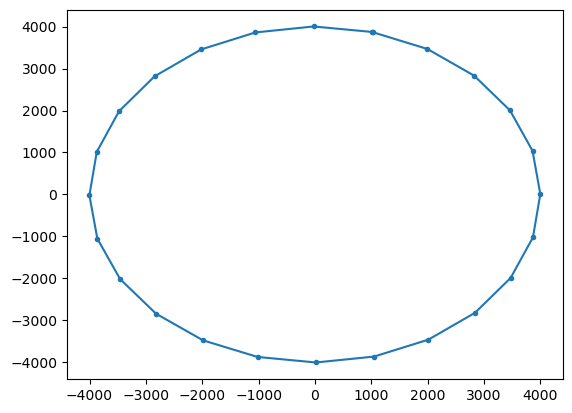While Lagrangian Ocean Analysis has been around since at least the 1980s, the Blanke and Raynaud (1997) paper has really spurred the use of Lagrangian particles for large-scale simulations. In their 1997 paper, Blanke and Raynaud introduce the so-called Analytical Advection scheme for pathway integration. This scheme has been the base for the Ariane and TRACMASS tools. We have also implemented it in Parcels, particularly to facilitate comparison with for example the Runge-Kutta integration scheme.

In this tutorial, we will briefly explain what the scheme is and how it can be used in Parcels. For more information, see for example Döös et al (2017).

Note that the Analytical scheme works with a few limitations:

1. The velocity field should be defined on a C-grid (see also the Parcels NEMO tutorial).

And specifically for the implementation in Parcels

1. The AdvectionAnalytical kernel only works for Scipy Particles.

2. Since Analytical Advection does not use timestepping, the dt parameter in pset.execute() should be set to np.inf. For backward-in-time simulations, it should be set to -np.inf.

3. For time-varying fields, only the ‘intermediate timesteps’ scheme (section 2.3 of Döös et al 2017) is implemented. While there is also a way to also analytically solve the time-evolving fields (section 2.4 of Döös et al 2017), this is not yet implemented in Parcels.

We welcome contributions to the further development of this algorithm and in particular the analytical time-varying case. See here for the code of the AdvectionAnalytical kernel.

Below, we will show how this AdvectionAnalytical kernel performs on one idealised time-constant flow and two idealised time-varying flows: a radial rotation, the time-varying double-gyre as implemented in e.g. Froyland and Padberg (2009) and the Bickley Jet as implemented in e.g. Hadjighasem et al (2017).

First import the relevant modules.

:

from datetime import timedelta as delta

import matplotlib.pyplot as plt
import numpy as np
import xarray as xr
from IPython.display import HTML
from matplotlib.animation import FuncAnimation

from parcels import (
FieldSet,
JITParticle,
ParticleSet,
ScipyParticle,
Variable,
)


As in Figure 4a of Lange and Van Sebille (2017), we define a circular flow with period 24 hours, on a C-grid

:

def radialrotation_fieldset(xdim=201, ydim=201):
# Coordinates of the test fieldset (on C-grid in m)
a = b = 20000  # domain size
lon = np.linspace(-a / 2, a / 2, xdim, dtype=np.float32)
lat = np.linspace(-b / 2, b / 2, ydim, dtype=np.float32)
dx, dy = lon - lon, lat - lat

# Define arrays R (radius), U (zonal velocity) and V (meridional velocity)
U = np.zeros((lat.size, lon.size), dtype=np.float32)
V = np.zeros((lat.size, lon.size), dtype=np.float32)
R = np.zeros((lat.size, lon.size), dtype=np.float32)

def calc_r_phi(ln, lt):
return np.sqrt(ln**2 + lt**2), np.arctan2(ln, lt)

omega = 2 * np.pi / delta(days=1).total_seconds()
for i in range(lon.size):
for j in range(lat.size):
r, phi = calc_r_phi(lon[i], lat[j])
R[j, i] = r
r, phi = calc_r_phi(lon[i] - dx / 2, lat[j])
V[j, i] = -omega * r * np.sin(phi)
r, phi = calc_r_phi(lon[i], lat[j] - dy / 2)
U[j, i] = omega * r * np.cos(phi)

data = {"U": U, "V": V, "R": R}
dimensions = {"lon": lon, "lat": lat}
fieldset = FieldSet.from_data(data, dimensions, mesh="flat")
fieldset.U.interp_method = "cgrid_velocity"
fieldset.V.interp_method = "cgrid_velocity"
return fieldset



Now simulate a set of particles on this fieldset, using the AdvectionAnalytical kernel. Keep track of how the radius of the Particle trajectory changes during the run.

:

def UpdateR(particle, fieldset, time):
particle.radius = fieldset.R[time, particle.depth, particle.lat, particle.lon]

class MyParticle(ScipyParticle):

pset = ParticleSet(fieldsetRR, pclass=MyParticle, lon=0, lat=4e3, time=0)

pset.execute(
runtime=delta(hours=24),
dt=np.inf,  # needs to be set to np.inf for Analytical Advection
output_file=output,
)

WARNING: Particle initialisation from field can be very slow as it is computed in scipy mode.


Now plot the trajectory and calculate how much the radius has changed during the run.

:

ds = xr.open_zarr("radialAnalytical.zarr")
plt.plot(ds.lon.T, ds.lat.T, ".-")


Particle radius at start of run 4000.0
Particle radius at end of run 4002.48388671875## Double-gyre example#

Define a double gyre fieldset that varies in time

:

def doublegyre_fieldset(times, xdim=51, ydim=51):
"""Implemented following Froyland and Padberg (2009)
10.1016/j.physd.2009.03.002"""
A = 0.25
delta = 0.25
omega = 2 * np.pi

a, b = 2, 1  # domain size
lon = np.linspace(0, a, xdim, dtype=np.float32)
lat = np.linspace(0, b, ydim, dtype=np.float32)
dx, dy = lon - lon, lat - lat

U = np.zeros((times.size, lat.size, lon.size), dtype=np.float32)
V = np.zeros((times.size, lat.size, lon.size), dtype=np.float32)

for i in range(lon.size):
for j in range(lat.size):
x1 = lon[i] - dx / 2
x2 = lat[j] - dy / 2
for t in range(len(times)):
time = times[t]
f = (
delta * np.sin(omega * time) * x1**2
+ (1 - 2 * delta * np.sin(omega * time)) * x1
)
U[t, j, i] = -np.pi * A * np.sin(np.pi * f) * np.cos(np.pi * x2)
V[t, j, i] = (
np.pi
* A
* np.cos(np.pi * f)
* np.sin(np.pi * x2)
* (
2 * delta * np.sin(omega * time) * x1
+ 1
- 2 * delta * np.sin(omega * time)
)
)

data = {"U": U, "V": V}
dimensions = {"lon": lon, "lat": lat, "time": times}
allow_time_extrapolation = True if len(times) == 1 else False
fieldset = FieldSet.from_data(
data, dimensions, mesh="flat", allow_time_extrapolation=allow_time_extrapolation
)
fieldset.U.interp_method = "cgrid_velocity"
fieldset.V.interp_method = "cgrid_velocity"
return fieldset

fieldsetDG = doublegyre_fieldset(times=np.arange(0, 3.1, 0.1))


Now simulate a set of particles on this fieldset, using the AdvectionAnalytical kernel

:

X, Y = np.meshgrid(np.arange(0.15, 1.85, 0.1), np.arange(0.15, 0.85, 0.1))

psetAA = ParticleSet(fieldsetDG, pclass=ScipyParticle, lon=X, lat=Y)

output = psetAA.ParticleFile(name="doublegyreAA.zarr", outputdt=0.1)

psetAA.execute(
dt=np.inf,  # needs to be set to np.inf for Analytical Advection
runtime=3,
output_file=output,
)


And then show the particle trajectories in an animation

:

%%capture

ds = xr.open_zarr("doublegyreAA.zarr")

fig = plt.figure(figsize=(7, 5), constrained_layout=True)

ax.set_ylabel("Meridional distance [m]")
ax.set_xlabel("Zonal distance [m]")
ax.set_xlim(0, 2)
ax.set_ylim(0, 1)

timerange = np.unique(ds["time"].values[np.isfinite(ds["time"])])

# Indices of the data where time = 0
time_id = np.where(ds["time"] == timerange)

sc = ax.scatter(ds["lon"].values[time_id], ds["lat"].values[time_id], c="b")

t = str(timerange.astype("timedelta64[ms]"))
title = ax.set_title(f"Particles at t = {t}")

def animate(i):
t = str(timerange[i].astype("timedelta64[ms]"))
title.set_text(f"Particles at t = {t}")

time_id = np.where(ds["time"] == timerange[i])
sc.set_offsets(np.c_[ds["lon"].values[time_id], ds["lat"].values[time_id]])

anim = FuncAnimation(fig, animate, frames=len(timerange), interval=100)

:

HTML(anim.to_jshtml())

: